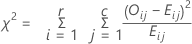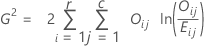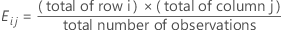# Methods and formulas for Chi-Square Test for Association

Select the method or formula of your choice.

## Chi-square test statistics

Minitab displays two chi-square statistics.

Pearson chi-square statistic:Likelihood-ratio chi-square statistic:###### Note

If any cell has an expected frequency less than one, the p-value for the test is not displayed because the results may not be valid. If some cells have small expected frequencies, consider combining or omitting row and/or column categories.

Yates' correction for 2 x 2 tables is not used.

### Notation

TermDescription
Oijobserved frequency in cell (i, j)
Eij

expected frequency for cell (i, j)r number of rows
c number of columns
The degrees of freedom associated with a contingency table possessing r rows and c columns equals (r – 1)(c – 1).

## Expected cell count

The expected count is the frequency that would be expected in a cell, on average, if the variables are independent. Minitab calculates the expected counts as the product of the row and column totals, divided by the total number of observations.

### Notation

TermDescription
ni+ number of observations in the ith row
n+j number of observations in the jth column
n++ total number of observations

## Raw residual calculation

### Formula

Raw residual = Oij – Eij

### Notation

TermDescription
Oij observed frequency in cell (i, j)
Eij expected frequency for cell (i, j)

## Standardized residual calculation

### Notation

TermDescription
Oij observed frequency in cell (i, j)
Eij expected frequency for cell (i, j)

### Notation

TermDescription
Oij observed frequency in cell (i, j)
Eij expected frequency for cell (i, j)
ni+ number of observations in the ith row
n+j number of observations in the jth column
n++ total number of observations

## Contribution to chi-square statistic from each cell

### Formula

The contribution to the χ2 statistic from each cell is:

### Notation

TermDescription
Oij observed frequency in cell (i, j)
Eij expected frequency in cell (i, j)
By using this site you agree to the use of cookies for analytics and personalized content.  Read our policy